A generalization of the concept of a solvable group. Letbe a certain set of prime numbers. A finite group for which the order of each composition factor either is coprime to any member ofor coincides with a certain prime in, is called a-solvable group. The basic properties of-solvable groups are similar to the properties of solvable groups. A-solvable group is a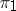-solvable group for any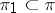; the subgroups, quotient groups and extensions of a-solvable group by a-solvable group are also-solvable groups. In a-solvable groupevery-subgroup (that is, a subgroup all prime factors of the order of which belong to) is contained in some Hall-subgroup (a Hall-subgroup is one with index in the group not divisible by any prime in) and every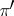-subgroup (whereis the complement ofin the set of all prime numbers) is contained in some Hall-subgroup; all Hall-subgroups and also all Hall-subgroups are conjugate in; the index of a maximal subgroup of the groupis either not divisible by any number inor is a power of one of the numbers of the set(see ). The number of Hall-subgroup inis equal to, where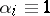(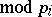) for every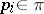which divides the order of, and, moreover,divides the order of one of the chief factors of(see ).